# 1 Preface

These are some (extremely sketchy) notes I’ve taken while attending the last part of the program on K-theory, algebraic cycles and motivic homotopy theory at the Isaac Newton Institute (INI) in Cambridge. I don’t expect these will be even remotely useful to anyone else, but perhaps this will have some value to those who just want some vague idea of what topics were discussed in talks. If you find yourself perplexed by anything here, the fault is almost surely entirely my own, since I was likely perplexed myself when I wrote it in the first place. Moreover, if anything appears incorrect, that too is surely my fault, either due to my own misunderstandings, typesetting errors, or just not hearing things correctly. The moral of the story: abandon all hope, all ye who continue reading!

# 2 Talk 1: Tyler Kelly, Open Saito-Givental Invariants and Mirror Symmetry (Tuesday, July 19)

Realizations of cycles and motives play a role in the physics of amplitudes in string theory and the study of Feynman integrals. Today’s focus: mirror symmetry, with a focus on certain integrals over cycles. Goal of mirror symmetry: link

• Enumerative data for a symplectic manifold, algebraic variety, or complex function, or
• Periods of a so-called mirror

The ur-example is a quintic threefold.

How this example works: count degree $$d$$ rational curves in $$X \subset {\mathbb{P}}^4$$ a quintic threefold. The mirror is a crepant resolution of \begin{align*} Z(\sum x_i^5 - 5\psi \prod x_i ) \subseteq {\mathbb{P}}^4/(C_5)^3 ,\end{align*} a 1-parameter deformation of the quintic. Compute as some integral $$\int_\gamma \Omega_\psi$$.

How to construct the mirror? And how to distill the enumerative information?

Today’s goal: answer these questions for Landau-Ginzberg (LG) models $$(X,G,W)$$ where $$X$$ is a quasi-affine variety $$G\curvearrowright X$$ is a finite group, $$W = ?$$. These give mirrors to Fanos, and noncommutative symplectic deformations of complete intersections in toric varieties.

Example LG model: $$({\mathbb{C}}^n, \prod \mu_{r_i}, \sum x_i^{r_i}) \rightleftharpoons({\mathbb{C}}^n, 1, \sum x_i^{r_i})$$. Answers to these questions for LG models: the mirror is built via a combinatorial construction, which annoyingly isn’t geometric. For enumerative data: oscillatory integrals associated to the RHS using Saito-Givental theory the encapsulate FJRW invariants. Issue: deformation must be built perturbatively term-by-term.

## 2.1 Saito-Givental Theory in dimension 1

Start with $$W:{\mathbb{C}}^n\to {\mathbb{C}}$$ given by $$\sum x_i^{r_i}$$. Build a twisted de Rham complex where $$\delta = d + h^{-1}dW \vee({-})$$ to get $$0 \to \Omega^0 \xrightarrow{\delta} \cdots$$, then take hypercohomology. For e.g. $$W = x^r$$, a basis can be computed explicitly for $$H^1$$. One can cook up a perfect pairing between a certain relative homology $$H_n(X, \Re(W/H)\ll 0, {\mathbb{C}})$$ and this hypercohomology, roughly \begin{align*} (\psi, \omega) \mapsto \int_\xi e^{W\over h} \omega \end{align*} where $$\Re(W/h) \ll 0$$ so that the exponential term is small. Now take deformations to distill enumerative info from this periods. First approximation: take the versal deformation \begin{align*} W^s = \sum x_i^{r_i} + \sum s_{a_1,\cdots, a_n} \prod x_i^{a_i} .\end{align*} Integrate to get a power series whose coefficients are $$\psi_k \in {\mathbb{C}}{\left[\left[ s_{a_1,\cdots, a_n} \right]\right] }$$. Compute this using a dual basis and integration by parts.

Can try $$W = x^4 + s_2 s^2 + s_1 x + s_0$$. Problem: lack of linearity! But this can be fixed to obtain flat coordinates and a primitive form $$\omega$$.

Theorem: there is a deformed potential $$W^t$$ which has primitive form $$\omega = \,dx$$ and flat coordinates $$t_0, \cdots, t_{r-2}$$ for the Frobenius manifold construction via Saito-Givental theory for the LG model $$W = x^r$$. This is a generating function for open enumerative invariants.

Strategy: start with $$X$$, where we want to understand its enumerative geometry. Build the mirror $$\tilde X$$ using some type of open enumerative geometry, along with a deformation. Look at integrals of forms over pieces of $$\tilde X$$, and that should recover the enumerative counts.

Build a moduli of orbidisks $${\mathcal{M}}$$ by taking configurations of marked points on $${\mathbb{P}}^1$$, where points off of $${\mathbb{R}}$$ come in pairs of complex conjugates. The local picture at marked points: $$[{\mathbb{C}}/\mu_r]$$. Compactify by considering degenerations of configurations – internal nodes, boundary nodes, contracted boundaries: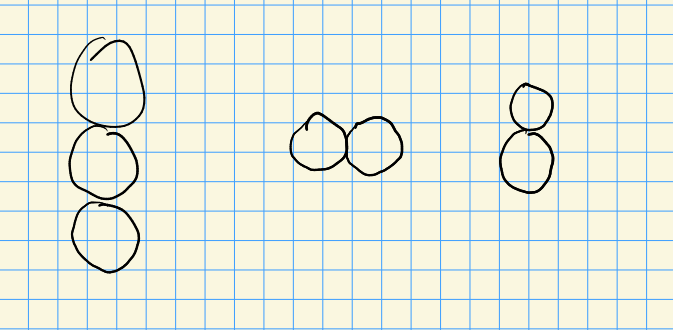The compactified moduli space is a real manifold with corners.

Take the universal line bundle $${\mathcal{L}}\to {\mathcal{M}}$$ and define the Witten bundle $$R^1(\pi_* {\mathcal{L}})^*$$. One needs to specify a canonical multisection $$s_{\mathrm{can}}$$ to control what happens on the boundary in order to integrate over $${\mathcal{M}}$$. Theorem: in dimension one, the open $$r{\hbox{-}}$$spin invariants exist and don’t depend on choice of this multisection, enjoy topological recursion, and there are closed form solutions of certain invariants. Parts of this theorem fail in dimension 2, although analogous statements can be made.

Open FJRW invariants exist for $$({\mathbb{C}}^2, \mu_r \times \mu_s, x^r+y^2)$$ and do depend on the choice of $$s_{\mathrm{can}}$$.

## 2.2 Dimension 2

Proceed as before, but run into issues with extra terms involving Gamma functions in $$h^0$$, as opposed to terms attached to $$h^{-1}$$ previously. A solution: insert a hypergeometric function to cancel it, as Li-Li-Saito-Shen do.

What’s the picture?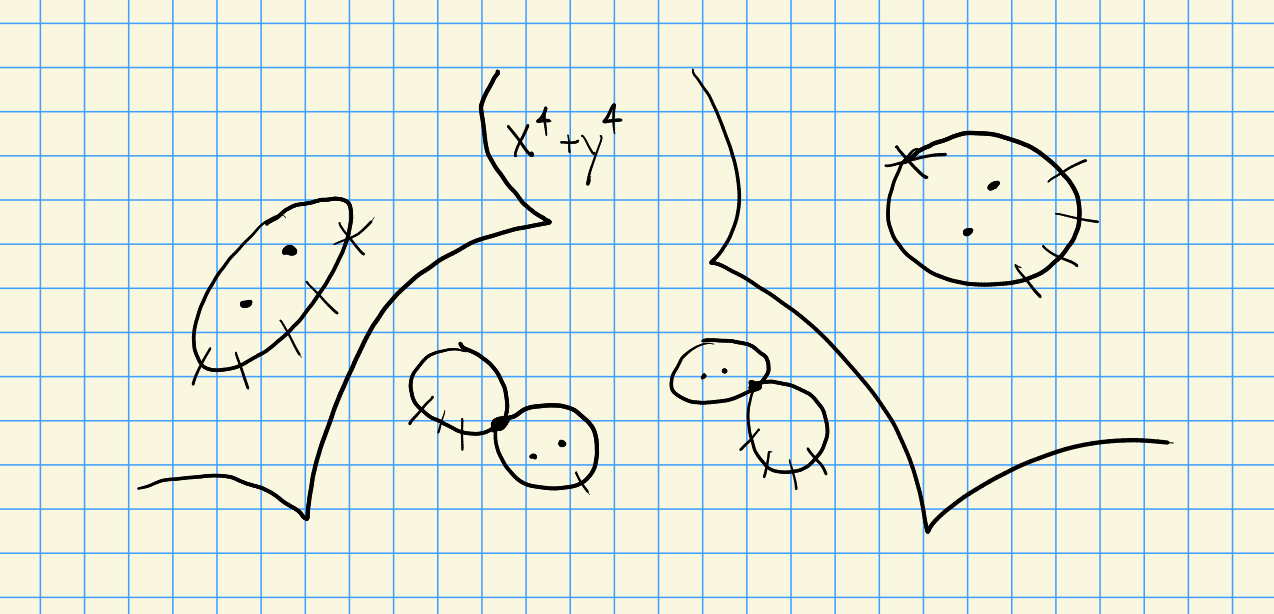There are some cases with mild singularities (ADE and elliptic type), outside of these things become unmanageable. Key foothold in the proof: no wall-crossing to deal with, which is advantageous. Proof uses topological recursion: compute something in two different ways, set them equal, and play the two formulae off of each other.

# 3 Talk 2: Man Wai Cheung, Cluster dualities and superpotentials for Grassmannian (Tuesday, July 19)

Goal: Marsh-Rietsch potential write down a potential for Grassmannians. Gross-Hacking-Keel-Kontsevich do as well, we’ll construct a map $$P$$ going from the former to the latter. Makes use of cluster structure on $${\operatorname{Gr}}$$, cluster varieties, and a GHKK construction.

Cluster algebras: start with a seed $$s = (\left\{{A_1,\cdots, A_n}\right\}, B = (b_{ij}))$$ where $$A_i$$ are variables and $$B = (b_{ij})$$ is a skew-symmetrizable matrix. Start with an initial seed, allow “mutations” taking $$s\to s'$$ where the new $$A_i$$ are functions of the old, and take all such possible iterations to generate the algebra.

Example: $$s = \qty{\left\{{A_1, A_2}\right\}, { \begin{bmatrix} {0} & {-1} \\ {1} & {0} \end{bmatrix} }}$$. Mutate at $$1$$ to get $$A_1 A_1' = 1 + A_2$$; write $$A_3 \mathrel{\vcenter{:}}= A_1'$$ to get $$A_3 = {1+A_2\over A_1}$$. The next seed is $$(\left\{{A_3, A_2}\right\}, B')$$.

Can express cluster structures in terms of triangulations of $$(n+3){\hbox{-}}$$gons: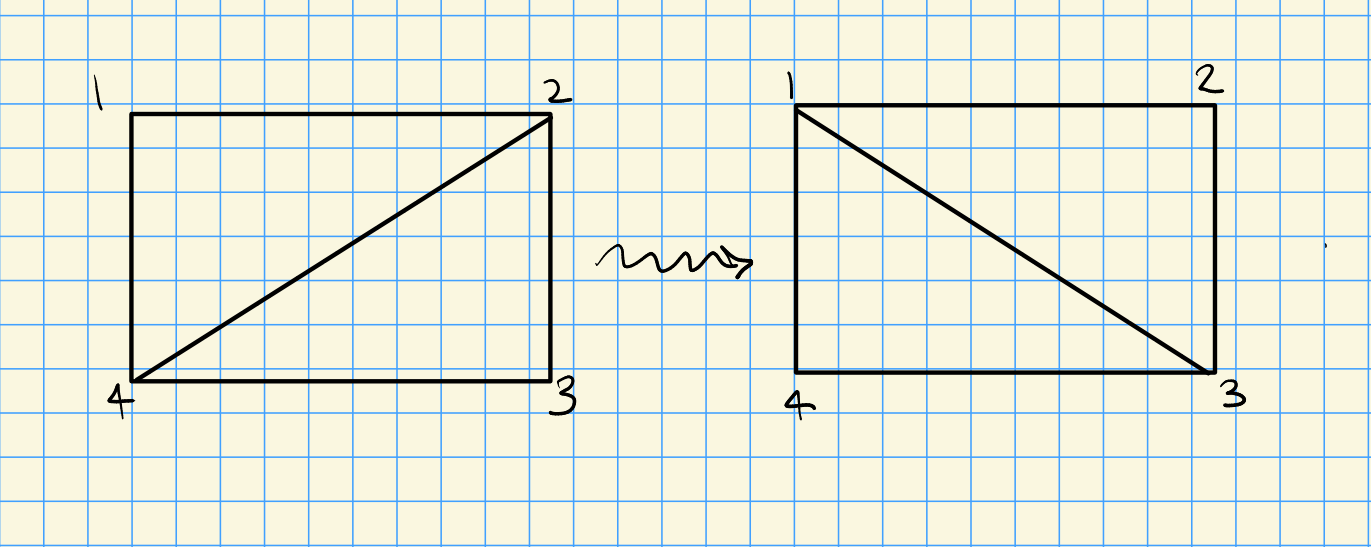This flip corresponds to the mutation relation $$A_{24} A_{13} = A_{12} A_{34} + A_{14} A_{23}$$. Since we can not flip the boundary, some variables are frozen – namely those corresponding to adjacent nodes, so $$A_{12}, A_{23}, A_{34}, A_{41}$$.

The homogeneous coordinate ring of $${\mathbb{C}}[{\operatorname{Gr}}_k(n)]$$ carries a cluster structure. On the geometry side, cluster varieties are log Calabi-Yau whose rings of regular functions carry cluster structures. For seeds, variables correspond to algebraic tori $$({\mathbb{C}}^{\times})^n$$, and mutations are birational maps between tori and defines a gluing between them.

Formally defining a cluster variety: start with a lattice $$N$$ and skew-symmetric form $$\left\{{{-}, {-}}\right\}$$ on $$N$$, and integers $$d_i$$, and a seed given by a basis for $$N$$. Take a dual basis $$M$$ and scale to $$M^\circ$$ by taking a basis $${1\over d_i} e_i^*$$. Take the corresponding tori $$A, X$$ and glue by mutations to get the corresponding cluster variety. The form gives a cluster ensemble lattice map $$p^*: N\to M^\circ$$ where $$n \mapsto \left\{{n, {-}}\right\}$$. Since these commute with mutation, and produce a map of varieties $$A\to X$$.

There is a Langlands dual given by sending $$B\mapsto B^{-t}$$. A conjecture (Bardwellcot-Evans-C-Hong-Lin) of mirror symmetry: $$A \rightleftharpoons X^L$$ and $$X\rightleftharpoons A^L$$. For today, the exchange matrices are skew-symmetric, so the expectation is that $$A \rightleftharpoons X$$ are mirrors.

Cluster varieties can be described by scattering diagrams: defining $$N_{\mathbb{R}}, M_{\mathbb{R}}$$, this is a collection of walls with finiteness and consistency conditions. A wall is a pair $$(d, f_d)$$ where $$d \subseteq M_{\mathbb{R}}$$ is the support of walls, a convex rational polyhedral cone of codimension 1.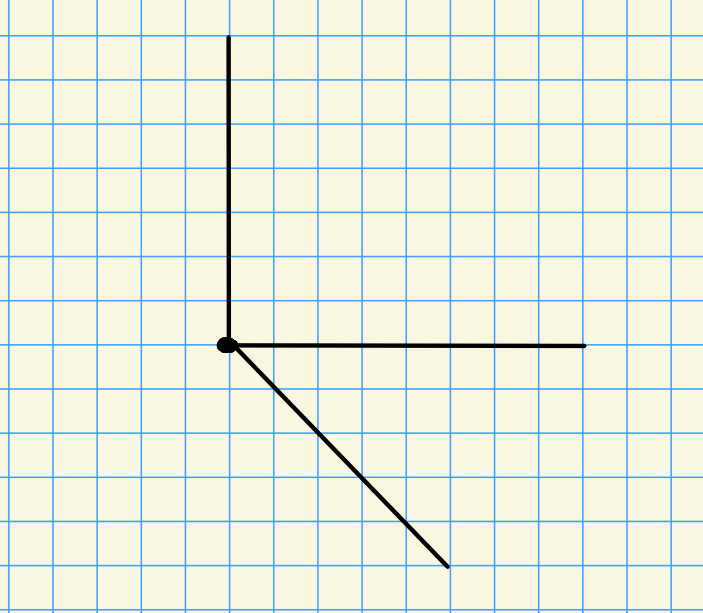Crossing walls: $$p_\gamma: z^m \mapsto z^m f_d^{\pm {\left\langle {n_0},~{m} \right\rangle} }$$ where $$n_0$$ is a primitive normal to a wall and $$\gamma$$ is a path between chambers. A motivating example for theta functions: $$({\mathbb{C}^{\times}})^2$$ satisfies $$H^0(({\mathbb{C}^{\times}})^2; {\mathcal{O}}) = \bigoplus _{m_1, m_2\in {\mathbb{Z}}} {\mathbb{C}}z_1^{m_1} z_2^{m_2}$$. To each nonzero point in $$M^\circ$$ assign a theta function $$\theta_m$$, defined from a collection of broken lines with initial slope $$m$$ and endpoint $$Q$$ in the positive chamber. Identify points in the scattering diagram with tropical points of Langlands dual cluster varieties.

Cluster structures for $${\operatorname{Gr}}$$: have a distinguished anticanonical divisor $$D_{\mathrm{ac} } = \sum _{i=1}^n D_i$$ where $$D_k = D_{[i+1, i+k]} = \left\{{p_{[i+1, i+k]} = 0}\right\}$$. Let $${\operatorname{Gr}}_k({\mathbb{C}}^n)^\circ = {\operatorname{Gr}}_k({\mathbb{C}}^n) / D$$, the positroid… something. Compactify using boundary divisors $$D_k = D_{[i+1, i+(n-k)]}$$. Each irreducible component yields a term in the potential of the mirror (something about LG models). Can somehow get valuations on the coordinate ring and write the potential directly.

Marsh-Rietsch define an isomorphism from the Jacobi ring of $$({\operatorname{Gr}}_k({\mathbb{C}}^n)^\circ, W)$$ to the equivariant quantum cohomology ring of $${\operatorname{Gr}}_{n-k}({\mathbb{C}}^n)$$. Rietsch-Williams: there is a Newton-Okounkov body on one side $$\Delta(D)$$, identifies points in this as tropical points on functions extending to the boundary of… a potential $$\Gamma$$? Use theta reciprocity to get $$\Delta(D) \cong \Gamma$$ via $$p^*$$.

# 4 Talk 3: Dani Kaufman, Cluster varieties, amplitude symbols, and mutation invariants (Tuesday, July 19)

## 4.1 Cluster Varieties

Recall: cluster varieties are varieties with a toric atlas generated by some extra combinatorial data. Encodes unexpected coordinate functions, and automorphisms of the cluster structure encode exotic automorphisms of the variety. Cluster structure for the Grassmannian: uses Plucker coordinates. New coordinate functions appear as part of a symbol alphabet for scattering amplitudes for $$N = 4$$ SYM (super Yang-Mills) theory.

Idea: the same function can be obtained from several different constructions, classifying mutation invariants shows that these must produce the same functions. Examples of such special functions: traces of monodromy, cluster character, Diophantine equations of Markov numbers, theta functions, Skein modules elements of closed loops, etc.

Cluster varieties: varieties built out of seed tori glued along birational morphisms called mutations.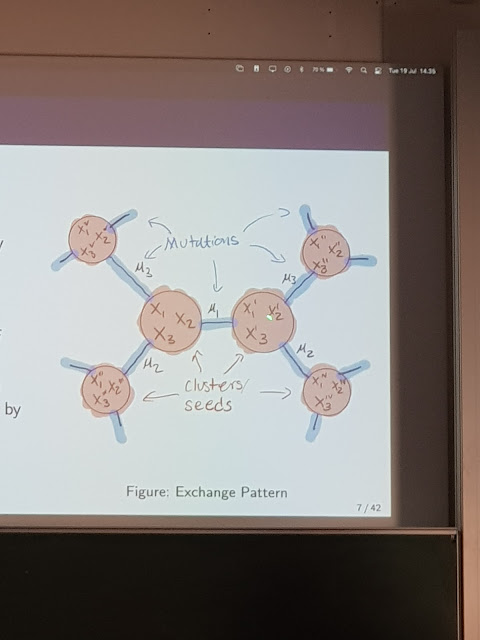Geometric type cluster varieties can be tracked with quivers – a quiver is used as the initial seed and generated a cluster variety after taking mutations. Cluster ensembles associated with a quiver $$Q$$: a pair of cluster varieties $$(A, X)$$ and a map $$p:A\to X$$ defined on coordinates on the same seed by $$p^*(x_i) = \prod_j a_j^{e_{ij}}$$. Introduced by Fock, Goncharov toward an algebraic theory of higher Teichmüller spaces. Example: $${\operatorname{Gr}}_p(p+q)$$ and $${\mathrm{Conf}}_n({\mathbb{P}}^{k-1})$$ are an $$(A, X)$$ pair. Interestingly, the immutable seeds for $${\operatorname{Gr}}_3(7)$$ look like an $$E_6$$ diagram! There are “phase transitions” for values of $$p,q$$ where there is a jump from finitely many clusters to infinitely many.

## 4.2 Amplitudes

Scattering amplitudes in the planar limit $$N=4$$ SYM live in $${\mathrm{Conf}}+n({\mathbb{P}}^3)$$, and the amplitudes for up to two loops can be written in terms of polylogarithms. The arguments of these polylogarithms come from the $$X$$ cluster variety structure on this configuration space, and constitute the symbol of the amplitude which encodes its singularity and branch cut structure. Goal: understand what happens as cluster ensembles transition from finite type to infinite type.

## 4.3 Cluster modular groups

Idea: automorphism group $$\Gamma$$ of mutation structures, so similar to a mapping class group. Can define an action of this on the rational function fields of these varieties. Mutation invariant functions: those invariant under some subgroup of $$\Gamma$$.

Example: take the Markov quiver, which is associated to the punctured torus and the affine root system of type $$A_1^{(1, 1)}$$ and $$\Gamma = {\operatorname{PSL}}_2({\mathbb{Z}})$$. Similarly a 4-punctured sphere is of type elliptic $$D_4^{(1, 1)}$$. See Somos sequences.

## 4.4 Surface cluster ensembles

Look at $$S$$ associated to a marked surface and take $$G = {\operatorname{PSL}}_2({\mathbb{R}})$$, then $$A$$ corresponds to Penner’s decorated Teichmüller spaces and $$X$$ with Teichmüller space with Fock coordinates parameterizing hyperbolic somethings with an “opening.”

Main theorem of their thesis: classification of invariants for the action of Dehn twists on surface cluster ensembles. The invariant ring $${\mathbb{R}}(X)^{\left\langle{\gamma}\right\rangle}$$ is generated by traces of monodromy operators of excised closed curves and invariant $$X$$ coordinates for an excising triangulation of $$\delta$$ a simple closed curve, where $$S$$ is a marked surface and $$\gamma\in \Gamma_S$$ is a Dehn twist about $$\delta$$. For $$A$$, it’s generated by curves that don’t intersect $$\delta$$.

There’s some way to obtain a quiver from a triangulation of a surface. More generally, each cluster modular group has an element that looks like a Dehn twist (a cluster Dehn twist), even when the cluster doesn’t come from a surface at all.

Some questions:

• What can we say about cluster invariants from higher Teichmüller spaces? Are these related to higher laminations, tensor webs?

• Are the square root symbols related to polylogarithm relations?

• The collection of invariants for a Dehn twist behave like cluster variables for a limit cluster ensemble. How is this limit related to compactifications of Teichmüller space?

# 5 Talk 4: Goncalo Tabuada, Noncommutative generalized Riemann hypothesis (Tuesday, July 19)

## 5.1 GRH

Recall $$\zeta(s) = \sum_{n\geq 1} {1\over n^s}$$, which admits an Euler product expansion $$\prod _{p\text{ prime}} {1\over 1-p^{-s}}$$, which converges for $$\Re(s) > 1$$ and has a simple pole at $$s=1$$.

\begin{align*} \zeta(s) = 0 \implies \Re(s) = {1\over 2} .\end{align*}

Formulating GRH: let

• $$K$$ be a global field (e.g. $$K={\mathbb{Q}}$$), so for $$\operatorname{ch}(K) = 0$$ a finite extension of $${\mathbb{Q}}$$ and for $$\operatorname{ch}(K) > 0$$ an extension of $${\mathbb{F}}_q(t)$$.
• $$X$$ be a smooth proper $$K{\hbox{-}}$$scheme.
• $$0\leq w \leq 2\dim(X)$$
• $$\Sigma_K$$ the infinite set of nonarchimedean places of $$K$$
• For $$v\in \Sigma_k$$,
• $$K_V$$ the completion of $$K$$ at $$v$$ (e.g. $${ {\mathbb{Q}}_p }$$)
• $${\mathcal{O}}_v$$ the valuation ring of $$K_V$$ (e.g. $${ {\mathbb{Z}}_{\widehat{p}} }$$)
• $$\kappa_v$$ the residue field of $${\mathcal{O}}_v$$ (e.g. $${\mathbb{Z}}/p{\mathbb{Z}}$$)
• $$p_v$$ the characteristic of $$\kappa_v$$ (e.g. $$p$$)
• $$N_v$$ the cardinality of $$\kappa_v$$ (e.g. $$p$$)

There is a SES \begin{align*} 1\to I_v \to G_{K_v} \to G_{\kappa_v} \cong \widehat{{\mathbb{Z}}}\to 1 \end{align*} where $$I_v$$ is the inertia group. Let the Frobenius be $$\pi_v$$, defined as the inverse of $$\lambda \mapsto \lambda^{N_v}\in \widehat{{\mathbb{Z}}}$$. Choose primes $$\ell_v$$ and field embeddings $$\iota_v: {\mathbb{Q}}_{\ell_v} \to {\mathbb{C}}$$.

\begin{align*} L_w(X; s) = \prod_{v\in \Sigma_k} L_{w, v}(X, s), \\ L_{w, v}(X; s) \mathrel{\vcenter{:}}={ 1 \over \operatorname{det}\qty{ \operatorname{id}- N_v^{-s} (\pi_v \otimes{\mathbb{C}}) \mathrel{\Big|} H_\text{ét}^w(X { \underset{\scriptscriptstyle {k} }{\times} } K_v { \underset{\scriptscriptstyle {K_v} }{\times} } \mkern 1.5mu\overline{\mkern-1.5muK_v\mkern-1.5mu}\mkern 1.5mu; {\mathbb{Q}}_{\ell_v} )^{I_v} \otimes{\mathbb{C}} } } .\end{align*}

$$L_w(X; s)$$ is a meromorphic function on the half-plane $$\Re(s) > {w\over 2} + 1$$. Some conditions:

• $$L_w(X; s)$$ admits a meromorphic continuation to $${\mathbb{C}}$$
• $$L_w(X; s)$$ has no poles in the critical strip $${w\over 2}<\Re(s) < {w\over 2} + 1$$.

$$L_w(X; s) = 0$$ and $${w\over 2} < \Re(s) < {w\over 2} + 1 \implies \Re(s) = {w+1\over 2}.$$

For $$K = {\mathbb{Q}}, X = \operatorname{Spec}{\mathbb{Q}}, w=0$$ then $$L_0(X; s) = \zeta(s)$$.

For $$K$$ a number field and $$X = \operatorname{Spec}K$$, $$L_0(X; s) = \sum_{I{~\trianglelefteq~}{\mathcal{O}}_K} {1\over N(I)^s}$$ is the Dedekind-zeta function.

Status of GRH:

• For $$\operatorname{ch}k = 0$$, no cases are known. The case of a point $$\operatorname{Spec}{\mathbb{Q}}$$ is already classical RH!

• $$\operatorname{ch}k > 0$$ follows from Grothendieck-Deligne.

## 5.2 Noncommutative geometry

Recall that a DG-category is a category enriched over complexes of $$K{\hbox{-}}$$modules.

Examples: DG-algebras and $$\mathsf{Perf}_{{\mathsf{dg}}}(X)$$ for $$X\in {\mathsf{Sch}}_{/ {K}}$$. By Beilinson, $$X = {\mathbb{P}}^1$$, $$\mathsf{Perf}_{{\mathsf{dg}}}({\mathbb{P}}^1)$$ is Morita-equivalent to $${ \begin{bmatrix} {K} & {K \oplus K} \\ {0} & {K} \end{bmatrix} }$$.

Let $$\mathsf{A}$$ be a DG-category, and say $$\mathsf{A}$$ is a geometric NC scheme if there exists a smooth proper $$X\in {\mathsf{Sch}}_{/ {K}}$$ such that there is an admissible embedding $$A \hookrightarrow\mathsf{Perf}_{\mathsf{dg}}(X)$$.

$$A$$ a finite dimensional $$k{\hbox{-}}$$algebra with finite global dimension where $$A/K$$ is $$K{\hbox{-}}$$ separable defines a geometric NC scheme. For the quiver $$Q \mathrel{\vcenter{:}}=(\cdot \to \cdot)$$, $$KQ = { \begin{bmatrix} {K} & {K} \\ {0} & {K} \end{bmatrix} }$$ is not Morita-equivalent to $$\mathsf{Perf}_{\mathsf{dg}}(Y)$$ for any smooth proper $$Y$$. So there exist NC schemes which are not themselves schemes.

Can GRH be formulated in the NC setting? Attempt to define NC $$\ell{\hbox{-}}$$adic cohomology: let

• $$K$$ be a field
• $$\ell \neq \operatorname{ch}(K)$$ a prime
• $$A$$ a geometric NC scheme

Define the étale $${\mathsf{K}}{\hbox{-}}$$theory of $$A$$ as \begin{align*} {\mathsf{K}}^\text{ét}(A)_{\widehat{\ell}} \mathrel{\vcenter{:}}=\mathop{\mathrm{hocolim}}_{n\geq 0} L_{{\operatorname{KU}}} {\mathsf{K}}(A; {\mathbb{Z}}/\ell^n) .\end{align*} Define \begin{align*} \begin{cases} H_{\text{even}, \ell}(A) & \pi_0 {\mathsf{K}}^\text{ét}( (A\otimes_K \mkern 1.5mu\overline{\mkern-1.5muK\mkern-1.5mu}\mkern 1.5mu)_{\widehat{\ell}} ) \otimes_{\mathbb{Z}}{\mathbb{Z}}{ \left[ { \scriptstyle \frac{1}{\ell} } \right] } \\ H_{\text{odd}, \ell}(A) & \pi_1 {\mathsf{K}}^\text{ét}( (A\otimes_K \mkern 1.5mu\overline{\mkern-1.5muK\mkern-1.5mu}\mkern 1.5mu)_{\widehat{\ell}} ) \otimes_{\mathbb{Z}}{\mathbb{Z}}{ \left[ { \scriptstyle \frac{1}{\ell} } \right] }. \end{cases} \end{align*} These are $${ {\mathbb{Q}}_\ell }{\hbox{-}}$$linear $$G_{K}{\hbox{-}}$$modules, and can be used to define NC $$L{\hbox{-}}$$functions.

Let $$K$$ be a global field, $$A$$ a NC geometric $$K{\hbox{-}}$$scheme. Define \begin{align*} L_\text{even}(A, s) \mathrel{\vcenter{:}}=\prod_{v\in \Sigma_K} L_{\text{even}, v}(A; s) \\ L_{\text{even}, v}(A; s) = {1 \over \operatorname{det}\qty{ \operatorname{id}- N_v^{-s} (\pi_v \otimes{\mathbb{C}}) \mathrel{\Big|} H_{\text{even}, \ell_v} (A\otimes_K K_v)^I_V \otimes{\mathbb{C}} } } ,\end{align*} and $$L_{\text{odd}, v}$$ similarly.

$$L_{\text{even}}(A; s)$$ is a meromorphic function on $$\Re(s) > 1/2$$, and odd for $$\Re(s) > 3/2$$.

The even zeros in the critical strip are on $$\Re(s) = {1\over 2}$$, and the odd ones on $$\Re(s) = 1$$.

Let $$X$$ be a smooth proper $$K{\hbox{-}}$$scheme. Then $$R_\text{even}(\mathsf{Perf}_{\mathsf{dg}}(X)) \iff \left\{{R_w(X)}\right\}_{w \text{ even}}$$, and similarly for odd, where $$R$$ denotes the various forms of GRH.

## 5.3 Applications to commutative geometry

Let $$K$$ be a global field with $$\operatorname{ch}(K) = 0$$ and let $$X, Y$$ be smooth proper $$K{\hbox{-}}$$schemes and assume condition (C2). Suppose $$\mathsf{Perf}(X) \cong \mathsf{Perf}(Y)$$ is a Fourier-Mukai equivalence, then $$\left\{{R_w(X)}\right\}_w \iff \left\{{R_w(Y)}\right\}_{w}$$. So this version of GRH is invariant under derived equivalence.

Suppose $$X$$ is homologically projectively dual (HPD) to $$Y$$, with $$X\to {\mathbb{P}}(V)$$ and $$Y\to {\mathbb{P}}(V {}^{ \vee })$$. For $$L \subseteq V {}^{ \vee }$$, write $$X_L \mathrel{\vcenter{:}}= X{ \underset{\scriptscriptstyle {{\mathbb{P}}(V)} }{\times} } {\mathbb{P}}(L^\perp)$$ and $$Y_L = Y{ \underset{\scriptscriptstyle {{\mathbb{P}}(V {}^{ \vee })} }{\times} } {\mathbb{P}}(L)$$.

See

• Veronese-Clifford duality
• Grassmannian-Pfaffian duality
• Spinor duality?
• Determinantal duality?

Let $$V_1 \cdots V_n$$ be simple $$A/J{\hbox{-}}$$modules and consider $$\mathop{\mathrm{End}}(V_i)$$ which are division $$K{\hbox{-}}$$algebras. Then $$K \hookrightarrow K_i \hookrightarrow\mathop{\mathrm{End}}(V_i)$$ where the $$K_i$$ are finite separable extensions, and \begin{align*} \left\{{R_0(\operatorname{Spec}K_i)}\right\}_{1\leq i\leq m} \implies R_\text{even}(A) .\end{align*}

This doesn’t exactly help prove GRH, but does help establish properties of various conjectures. See paper of Thomason for paper on étale $${\mathsf{K}}{\hbox{-}}$$theory.

Some analogs: $$H_\mathrm{dR}\mapsto HP$$, $$H_{{\mathrm{crys}}} \mapsto TP$$ (topological periodic cyclic homology) which comes equipped with a Frobenius and can be used to define zeta functions.

# 6 Herbert Gangl, The Aomoto polylogarithm via iterated integrals (Wednesday, July 20)

The plan:

• Iterated integrals and formal versions, leading to the “algebraic fingerprint” (i.e. symbols).
• Aomoto polylogarithms
• A new choice of coordinates on configuration spaces
• Relating Aomoto polylogarithms to iterated integrals via the symbol

## 6.1 Classical Polylogarithms

Recall \begin{align*} \mathrm{Li}_n(z) = \sum_{k\geq 1} {z^k\over k^n } .\end{align*} Here $$n$$ is a weight, and the depth is 1 (the number of variables). More generally, for example, $$\mathrm{Li}_{m, n}(x, y)$$ has weight $$m+n$$ and depth 2. This can be written as an iterated integral: \begin{align*} \mathrm{II}(a_0; a_1,\cdots, a_n: a_{n+1}) = \int_{\Delta_\gamma} {dt_1 \over t_1-a_1} \wedge \cdots\wedge {dt_n \over t_n - a_n} \end{align*} where $$\gamma: [0, 1]\to {\mathbb{C}}\setminus\left\{{ a_1,\cdots, a_n }\right\}$$ with $$\gamma(0) = a_0, \gamma(1) = a_{n-1}$$ and $$\Delta_\gamma$$ is a certain simplex. Some properties:

• Form an algebra under the shuffle product
• Path composition
• Path reversal
• An associated differential equation

One can encode this formally as an algebra over a field $$F$$ with generators $$I(a_0, \cdots, a_{n+1})$$ and relations coming from the above properties. A new feature: a “semicircle coproduct” due to Goncharov, whose terms are indexed by polygons involving any subset of the $$a_i$$ when arranged along a semicircle: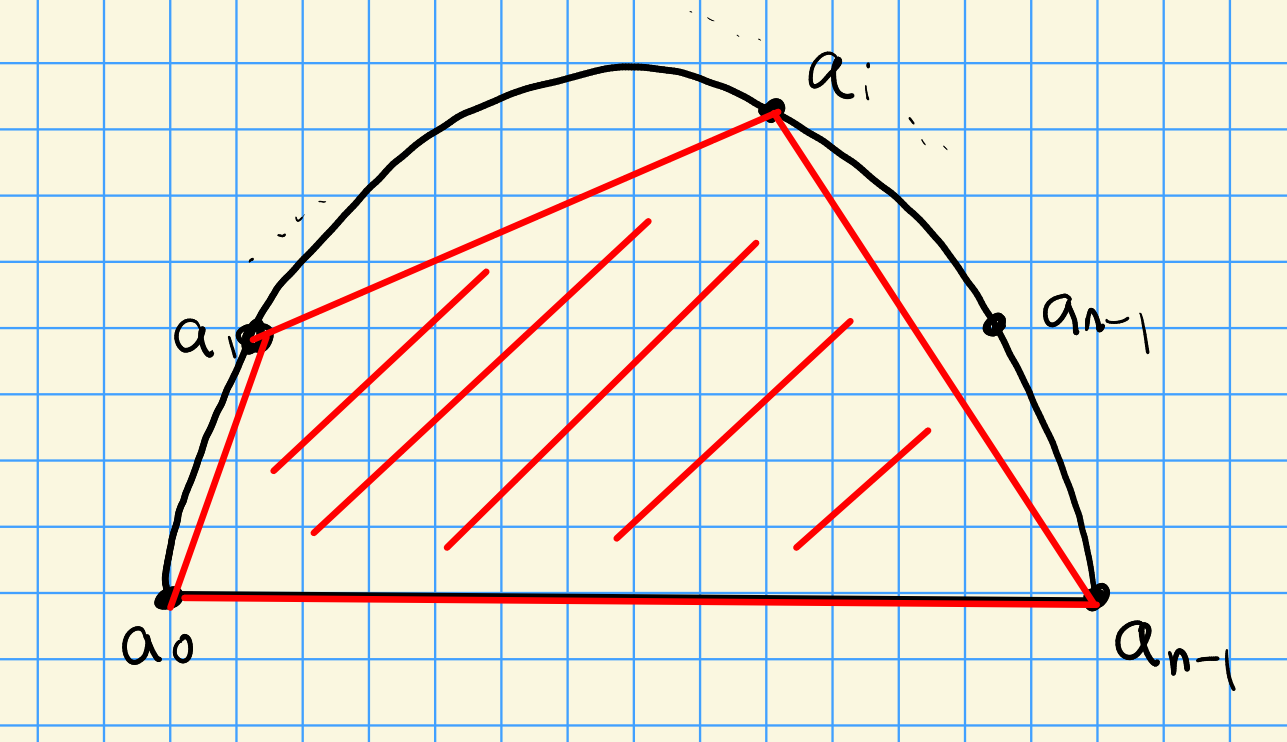In general, the symbol for $$I(\cdots)$$ will take values in $$(F^{\times}){ {}^{ \scriptstyle\otimes_{?}^{n} } }$$.

## 6.2 Aomoto Polylogarithms

Idea: attach a period to any pair of simplices $$(L, M)$$ in $${\mathbb{P}}^n({\mathbb{C}})$$ determined by coordinates $$(\ell_0,\cdots, \ell_n; m_0, \cdots, m_n)$$.

• To $$L$$, attach $$\omega_L$$ a differential form, $$\operatorname{dLog}\qty{z_1\over z_0} \wedge \cdots\wedge \operatorname{dLog}\qty{z_n\over z_0}$$.
• To $$M$$ attach an integral cycle.

This gives an assignment $$(L, M) \mapsto \int_{\Delta_M} \omega_L$$, which generalizes the classical polylogarithm. Some properties:

• Degeneracies go to zero (?)
• Skew-symmetry: $$(\sigma L, M) = (L, \sigma M) = \operatorname{sign}(\sigma)(L, M)$$ for any $$\sigma\in S_n$$.
• Invariance for $$g\in \operatorname{PGL}_{n+1}({\mathbb{C}})$$ in the sense that $$(gL, gM) = (L, M)$$.
• Additivity, a type of scissors congruence property: $$\sum_{i=0}^{n+1} (-1)^i (\ell_0, \cdots, \widehat{\ell}_i, \cdots, \ell_{n+1}; M ) = 0$$.

BGSV (’89) formalized this in an $$F{\hbox{-}}$$algebra with generators $$(L, M)$$ and relations coming from these new properties, and found a good candidate for the category of mixed Tate motives over a field. An issue is that the coproduct is only defined on generic such pairs.

Consider $$n=1$$ and $$(L, M)$$ with $$(\ell_0, \ell_1, m_0, m_1)$$, then \begin{align*} A_1(L, M) = \int_{m_0}^{m_1} \operatorname{dLog}\qty{z_1 - \ell_1 \over z_0 - \ell_0} .\end{align*}

One can extract a cross-ratio from this which lives in $$F^{\times}$$.

A surprise to BGSV: a certain expression can be written as a combination of Steinberg elements $$a\otimes(1-a)$$ which is related to motivic cohomology and the Bloch-Suslin complex. Goncharov also writes $$A_3(L, M)$$ in terms of polylogarithms, and attaches a symbol to general $$A_n(L, M)$$ in terms of Plucker coordinates.

The symbol of $$A_n(\mathbf{v})$$ can be written as a sum of iterated integrals.

# 7 Adriana Salerno and Ursula Whitcher, Diagonal pencils and Hasse-Witt invariants (Wednesday, July 20)

Calabi-Yau threefolds generalize elliptic curves, and K3s are somewhere in-between. Idea: use mirror symmetry to prove arithmetic properties of higher dimensional varieties, generalizing things we know about elliptic curves.

Consider the Legendre family $$X_\psi: y^2 = x(x-1)(x-\psi)$$ satisfies Picard-Fuchs, and over $${\mathbb{F}}_p$$ the trace of Frobenius is $$a_p = 1 + p - {\sharp}X_\psi({\mathbb{F}}_p)$$. Igusa noticed that the Picard-Fuchs equations for the holomorphic form is hypergeometric, and $$a_p$$ satisfies a truncated hypergeometric formula. Some progress on arithmetic properties of the Fermat quintic.

What is the mirror of smooth quintics in $${\mathbb{P}}^4$$? Start with the Fermat quintic pencil $$\sum x_i^5 - \psi \prod x_i = 0$$, which admits a $$C_5^3$$ action. Taking the quotient and resolving singularities yields the mirror $$Y$$. For $$\psi \in {\mathbb{Z}}$$, the point counts agree: $${\sharp}X({\mathbb{F}}_q) \cong {\sharp}Y({\mathbb{F}}_q)\operatorname{mod}q$$.

Generalized hypergeometric functions: \begin{align*} {}_A F_V(\alpha, \beta | z) = \sum_k {\alpha_{1, k} \cdots \alpha_{A, k} \over \beta_{1, k} \cdots \beta_{B, k}} z^k .\end{align*}

Daqing-Wang: for the Fermat pencil $$X_\psi$$ and its Greene-Plesser mirror $$Y_\psi$$, the unit roots of their zeta functions coincide, yielding the congruence of point counts. Kadir claims something similar works for generalize Fermat pencils of CYs in a Gorenstein Fano weighted projective space and its Greene-Plesser mirror. Recent work by (lots of people) uses BHK mirror symmetry to generalize to invertible pencils in $${\mathbb{P}}^n$$.

Batyrev’s construction: mirror families of CYs using reflexive polytopes. Look at polar polytopes, lattice polytopes $$\Delta$$ which contains $$0$$ in its interior. The facet equation can be normalized to $$\sum a_i x_i = -1$$, and there is an associated polar polytope $$\Delta^\circ$$ associated to the coefficients $$(a_i)$$. The polytope $$\Delta$$ is reflexive if $$\Delta^\circ$$ is again a lattice polytope. Note that there is no classification of reflexive polytopes in dimensions $$d\geq 5$$.

Building hypersurfaces: for each lattice point choose a parameter $$\alpha$$ and define $$p_\alpha = \sum_{M \cap} \alpha_m \prod x^{\cdots}$$. Can use this to somehow cook up mirror pairs of CYs? Using that CYs can be realized as hypersurfaces in toric varieties.

Kernel pairs: for $$\Delta, \Gamma$$ combinatorially equivalent polytopes, put vertices as columns of matrix and see if they have equal kernels. These are resolutions of quotients of a common toric variety, and the corresponding vertex pencils satisfy the same Picard-Fuchs equation.

An alternative to counting points for small primes: for $$X$$ a smooth variety, Frobenius induces a $$p{\hbox{-}}$$linear operator $$H^n(X; {\mathcal{O}}_X){\circlearrowleft}$$, and the matrix for this map in some basis is the Hasse-Witt matrix $$HW_p$$. Katz: for CYs, this determines the point counts mod $$p$$? A theorem of Huang-Lian-Yau-Yu shows that $$HW_p$$ for $$X$$ a Calabi-Yau satisfies a truncated relation and shows that the period integral has a certain power series expansion. For smooth CYs, we have a good idea of what $$HW_p$$ is.

Idea: for mirror kernel pairs, can sometimes replace a very complicated equation with a much simpler equation for its mirror, e.g. if one wants to do point counts or study the Picard-Fuchs equation. Can classify K3 surfaces by their $$HW_p$$.

Picard-Fuchs for rank 19 lattice-polarized K3s can be written as the symmetric square of a 2nd order linear Fuchsian differential equation. For hypergeometric functions, can use Clausen’s formula.

Let $$\Gamma \subseteq {\operatorname{PSL}}_2({\mathbb{R}})$$, and define a modular curve as $$\mkern 1.5mu\overline{\mkern-1.5mu\dcosetl{\Gamma}{{\mathbb{H}}}\mkern-1.5mu}\mkern 1.5mu$$. See Atkin-Lehner map. Group I in the classification is related to Monstrous Moonshine, and the classification shows it’s associated to $$\Gamma_0(3)^+$$ where plus means retaining all Atkin-Lehner involutions.

# 8 Daniel Kaplan, Quiver varieties and symplectic resolutions of singularities (Wednesday, July 20)

Three main sections:

• Spaces you (yes you!) care about can be realized as quiver varieties,
• These are well-understood with respect to symplectic resolutions,
• (joint work with Travis Schedler) Even more spaces are “locally” quiver varieties.

Let $$C_2\curvearrowright{\mathbb{C}}^2$$ by negation, then $$C^2\curvearrowright{\mathbb{C}}[{\mathbb{C}}^2] = {\mathbb{C}}[x, y]$$ by $$f(x, y) \mapsto f(-x, -y)$$. The invariants are $$R\mathrel{\vcenter{:}}={\mathbb{C}}[x, y]^{C_2} = {\mathbb{C}}[x^2, y^2, xy] = {\mathbb{C}}[y,v,w]/\left\langle{uv-w^2}\right\rangle$$ which is a type $$A_1$$ singularity at the origin. Take a blowup $$\operatorname{Bl}_0(X) \to X \mathrel{\vcenter{:}}=\operatorname{Spec}R$$.

Let’s do the same thing but differently: consider the nilpotent cone $${\mathrm{Nil}}({\mathfrak{sl}}_2) = \left\{{m = { \begin{bmatrix} {a} & {b} \\ {c} & {d} \end{bmatrix} } {~\mathrel{\Big\vert}~}{\mathrm{tr}}(m) = \operatorname{det}(m) = 0}\right\} = \left\{{{ \begin{bmatrix} {a} & {b} \\ {c} & {d} \end{bmatrix} } {~\mathrel{\Big\vert}~}a^2-bc=0}\right\}$$, which is the same equation as before. Take pairs $$(m, \ell\in \ker m)$$, then the projection map $$(m, \ell) \mapsto m$$ is the cotangent bundle $${\mathbf{T}} {}^{ \vee }{\mathbb{P}}^1\to {\mathrm{Nil}}({\mathfrak{sl}}_2)$$. Now perhaps it’s clear how to generalize, e.g. by taking other lie algebras.

## 8.1 Quivers

A quiver is the data $$Q = (Q_0, Q_1, s,t: Q_1\to Q_0)$$ where $$Q_0$$ is a vertex set and $$Q_1$$ is an arrow set, and everything is over a field $$k={\mathbb{C}}$$ in our case. Fix $$d\in {\mathbb{N}}^{Q_0}$$, a tuple $$d = (d_i)_{i\in Q_0}$$, then \begin{align*} {\mathsf{Rep}}(Q, d) = \bigoplus _{q\in Q_1} \mathop{\mathrm{Hom}}_{{\mathsf{{\mathbb{C}}}{\hbox{-}}\mathsf{Mod}}}({\mathbb{C}}^{d_{s(q)} }, {\mathbb{C}}^{d_{t(q)} }) .\end{align*} There is an action $$G_d \mathrel{\vcenter{:}}=\prod_{i\in Q_0} \operatorname{GL}_{d_i}({\mathbb{C}})$$ by conjugation, which we sometimes quotient by.

The $$G_d$$ orbits are too big! Let $$A,B,C$$ be matrices and consider the quiver $${\mathbb{C}}^2 \xrightarrow{A} {\mathbb{C}}^2{\circlearrowleft}_{B} \xrightarrow{C} {\mathbb{C}}$$. It’s hard to find $$G_d{\hbox{-}}$$invariant functions, and in fact $${\mathbb{C}}[{\mathsf{Rep}}(Q, d)]^{G_d} = {\mathbb{C}}[\left\{{ {\mathrm{tr}}(\alpha), \alpha \text{ a cycle} }\right\} ]$$. Note that $$B$$ is a cycle in this case.

There is a process of doubling a quiver: add inverse arrows, and duplicate every loop: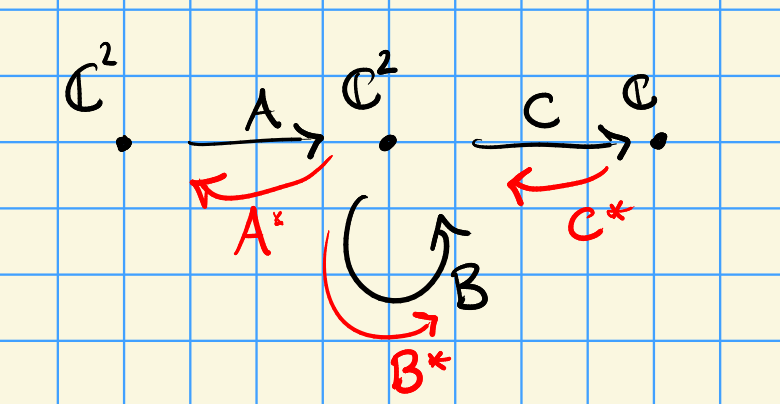Note \begin{align*} {\mathsf{Rep}}(\mkern 1.5mu\overline{\mkern-1.5muQ\mkern-1.5mu}\mkern 1.5mu, d) \to {\mathsf{Rep}}(Q, d) \oplus {\mathsf{Rep}}(Q^*, d) = {\mathsf{Rep}}(Q, d) \oplus {\mathsf{Rep}}(Q, d) {}^{ \vee }\to {\mathbf{T}} {}^{ \vee }{\mathsf{Rep}}(Q, d) ,\end{align*} which yields a cotangent bundle and puts us in the realm of symplectic geometry. The moment map is \begin{align*} \mu_d: {\mathsf{Rep}}(\mkern 1.5mu\overline{\mkern-1.5muQ\mkern-1.5mu}\mkern 1.5mu, d) \to {\mathfrak{g}}_d {}^{ \vee }&\leftarrow{\mathfrak{g}}_d \\ {\mathrm{tr}}(X({-})) &\mapsfrom X .\end{align*} Write $$\nu = \sum_{q\in Q_1} [q, q^*]$$ where the commutator is $$aa^* - a^*a$$, then $$p\in \mu_d^{-1}(0)$$ if $$p$$ is a module for the path algebra $${\mathbb{C}}\mkern 1.5mu\overline{\mkern-1.5mu{\mathbb{Q}}\mkern-1.5mu}\mkern 1.5mu/\left\langle{\nu}\right\rangle = \Pi(Q)$$.

Let $$\theta \in {\mathbb{Z}}^{Q_0} = \mathop{\mathrm{Hom}}_{{\mathsf{Alg}}{\mathsf{Grp}}}(\prod \operatorname{GL}_{d_i} ({\mathbb{C}}), {\mathbb{C}^{\times}})$$ where $$\theta \cdot \dim(\rho) = 0$$. Say $$\rho' \subseteq \rho$$ is destabilizing if $$\dim(\rho') \cdot \theta> 0$$, and $$\rho$$ is $$\theta{\hbox{-}}$$semistable if it does not contain any destabilizing $$\rho'$$. One can then define \begin{align*} {\mathcal{M}}(Q, d, \theta) = \mathop{\mathrm{Proj}}\bigoplus {\mathbb{C}}[ \mu_d^{-1}(0) ]^{k\theta ?} .\end{align*} This is an important object: the extra $$\theta$$ parameter can be sent to zero, and generically will yield a smooth space.

## 8.2 Local to global obstructions

There is a decomposition into symmetric spaces: \begin{align*} {\mathcal{M}}(Q, d, \theta) = \prod_{i = 1}^k \operatorname{Sym}^{n_i} {\mathcal{M}}(Q, d_i, \theta) \end{align*} where $$d = \sum n_i d_i$$ and $$d_i \cdot \theta = 0$$ for all $$i$$. Moreover, this has a symplectic resolution iff each factor does iff $$\gcd(d_i) = 1$$ or $$(\gcd(d_i), p\qty{d\over \gcd(d_i)} ) = (2, 2)$$.

Let $${\mathcal{M}}(A, d, \theta)$$ be the moduli space of $$\theta{\hbox{-}}$$semisimple $$A{\hbox{-}}$$modules of dimension $$d$$, where e.g. $$A = k\mkern 1.5mu\overline{\mkern-1.5muQ\mkern-1.5mu}\mkern 1.5mu / \left\langle{R}\right\rangle$$. There is an isomorphism of formal Poisson varieties after completing: \begin{align*} \widehat{{\mathcal{M}}}(\Lambda(Q), d, \theta)_m { \, \xrightarrow{\sim}\, }\widehat{{\mathcal{M}}}(Q', d', \theta)_0 ,\end{align*} where e.g. $$Q = Q'$$ in type $$\tilde A_n$$.

Local to global obstructions: since $$X$$ is Poisson, it has a stratification into symplectic leaves, $$X = {\textstyle\coprod}_i S_i$$. There is an action of $$\pi_1(S_i, x_i)$$ on the set of local resolutions $$\left\{{ \tilde U_{s_i} \xrightarrow{\pi} U_{s_i} }\right\}/W$$ where $$W$$ is a Nakajima-Weyl group. This action can be nontrivial, and there is a compatibility with leaf closures: if $$\mkern 1.5mu\overline{\mkern-1.5muS_i\mkern-1.5mu}\mkern 1.5mu \supseteq S_j$$ then $${ \left.{{ \pi_{\mkern 1.5mu\overline{\mkern-1.5muS\mkern-1.5mu}\mkern 1.5mu_i} }} \right|_{{S_j}} } = \pi_{S_j}$$.

In the situation $$C_2\curvearrowright({\mathbb{C}^{\times}})^2$$ there are four fixed points $$(\pm 1, \pm 1)$$ yielding simple singularities with local resolutions. The theorem implies there is a global symplectic resolution.

# 9 Jonathan Rosenberg, Twisted derived equivalences and string theory dualities with B-field (Wednesday, July 20)

Type II string theory: spacetime is $$X = {\mathbb{R}}^{10-2n} \times M$$ where $$M$$ is compact Kähler with a Ricci-flat metric and trivial canonical (the Calabi-Yau condition), not necessarily simply connected. For us: $$M$$ is smooth projective, $$X$$ is quasi-projective. The physics is controlled by D-branes (D for Dirichlet), either complex submanifolds in IIB or isotropic submanifolds for the symplectic structure in IIA. More generally, IIA branes are coherent sheaves, and IIA branes are classified by the Fukaya category. In type IIB, there is interest in $${ \mathsf{D} }^b {\mathsf{Coh}}(X)$$, since complexes can capture joining branes, separating, collapsing into other configurations, etc. There is a coarser topological classification given by topological $${\mathsf{K}}{\hbox{-}}$$theory (Minasian-Moore, Witten). The D-branes give classes in $${\operatorname{KU}}^0(M)$$ in IIB and $${\operatorname{KU}}^{-1}(M)$$ for IIA.

Today: orientifold string theories. Classically, strings moving in time yield maps $$\Sigma\to X$$ where $$\Sigma$$ is the string worldsheet and $$X$$ is spacetime. This is a $$\sigma{\hbox{-}}$$model. Orientifold theory: ask for strings to be equivariant with respect to involutions $$\iota$$ and worldsheet parity operators – if $$\iota = \operatorname{id}_X$$, this recovers type I string theory (forgetting string orientation). In this theory, D-brane charges live in real $${\mathsf{K}}{\hbox{-}}$$theory $${\mathsf{K}}R(X, \iota)$$, which reduces to $${\operatorname{KO}}(X)$$ when $$\iota = \operatorname{id}_X$$. 2015: joint work classifies orientifold string theories associated to (anti)holomorphic involutions on elliptic curves: there are 10. One case corresponds to Karoubi and Donovan’s twisted $${\mathsf{K}}{\hbox{-}}$$theory.

Can view an elliptic curve with antiholomorphic involution as a smooth genus one curve, possibly with no real points. Karoubi and Weibel (2003) show that $${\mathsf{K}}R$$ of the complex curve is related to $${\mathsf{K}}^\mathsf{Alg}$$ of the real variety. Suggests that duality of orientifold string theories may be reflected in a equivalence of derived categories for real (rather than complex) curves.

Derived equivalence: prototypical example is $${ \mathsf{D} }(X) \cong { \mathsf{D} }(Y)$$ for $$X,Y$$ smooth varieties over $$k$$ via Mukai duality. Formulated in terms of the Poincaré sheaf on $$X\times \widehat{X}$$ for $$X$$ an abelian variety and $$\widehat{X}$$ its dual. Integration over the fiber is really derived pushforward, so define the transform as \begin{align*} \phi({\mathcal{F}}) = {\mathbb{R}}\pi_{2, *} ({\mathcal{L}} \overset{\mathbb{L}}{ \otimes} \pi_{1}^* {\mathcal{F}}) .\end{align*} AVs are simple examples of CYs?

Elliptic curves are self-dual, so it works here, which suggests it may also work fiberwise for elliptic fibrations. Căldăraru considers elliptic fibrations $$f: X\to Z$$ with no section. The role of $$\widehat{X}$$ is the relative Jacobian $$\widehat{f}: J\to Z$$, the relative module space of semistable sheaves of rank 1 and degree zero on the fibers of $$f$$. Then $$\widehat{f}$$ is an elliptic fibration with section, but there’s no universal sheaf to play the role of the Poincaré sheaf $${\mathcal{L}}$$. Two reasons for this:

• $$J$$ may have singularities. Not so serious, can resolve and replace $$J$$ by a small resolution
• May exist locally but not globally.

It turns out that $${\mathcal{L}}$$ exists as a twisted sheaf: the transition functions may only agree up to a 2-cycle, defining a class $$\alpha$$ in the Brauer group $$H_\text{ét}^2(J; {\mathbb{G}}_m)$$. A similar recipe goes through: pull up to $$X{ \underset{\scriptscriptstyle {Z} }{\times} } J$$, tensor by the $$\alpha^{-1}{\hbox{-}}$$twisted sheaf $${\mathcal{L}}$$, and push down to $$X$$.

View a complex elliptic curve $$\mkern 1.5mu\overline{\mkern-1.5muE\mkern-1.5mu}\mkern 1.5mu$$ defined over $$\mkern 1.5mu\overline{\mkern-1.5mu{\mathbb{R}}\mkern-1.5mu}\mkern 1.5mu = {\mathbb{C}}$$ with a free antiholomorphic involution, regarded as $$C({\mathbb{C}})$$ with its $${ \mathsf{Gal}} ({\mathbb{C}}/{\mathbb{R}})$$ action for $$C$$ a smooth projective curve with $$g=1$$ defined over $${\mathbb{R}}$$ and $$C({\mathbb{R}}) = \emptyset$$. Think of $$C \to \operatorname{Spec}{\mathbb{R}}$$ as an elliptic fibration without a section, and the relative Jacobian as $$E\to \operatorname{Spec}{\mathbb{R}}$$. There is a Mukai equivalence $${ \mathsf{D} }(C) { \, \xrightarrow{\sim}\, }{ \mathsf{D} }(E, \alpha)$$ where $$\alpha\in \mathop{\mathrm{Br}}(E)/\mathop{\mathrm{Br}}({\mathbb{R}})$$ is the unique nonzero class.

This is an AG version of $$T{\hbox{-}}$$duality of orientifold theories, and $$\alpha$$ corresponds to the $$B{\hbox{-}}$$field.

## 9.1 Generalizations

Generalizing from genus 1 curves to K3s: let $$E_1, E_2$$ be elliptic curves over $${\mathbb{C}}$$ with real $$j{\hbox{-}}$$invariant, so they admit antiholomorphic involutions. Cook up a real structure on $$X = (E_1\times E_2)/\mu_2$$ and hence on its blowup $$\widehat{X}$$ which is a Kummer K3. Projection gives an elliptic fibration without a section. The relative Jacobian gives a dual $$K3$$ fibred over $${\mathbb{P}}^1$$ with a canonical section and the same derived equivalence as in the theorem. Can interpret as duality of orientifold type IIB string theories on K3s.

Generalizing to genus 1 curves over general fields: let $$\operatorname{ch}k = 0$$ and $$C_{/ {k}}$$ be a smooth connected projective curve with $$g=1$$ and let $$E$$ be its Jacobian. Then $$J$$ is elliptic over $$k$$ and $$C$$ is an $$E{\hbox{-}}$$torsor, so defines a class in $$\delta(C) \in H^1_\text{ét}(\operatorname{Spec}k, E(\mkern 1.5mu\overline{\mkern-1.5muk\mkern-1.5mu}\mkern 1.5mu))$$. Can how a $$d_2$$ vanishes in the Leray spectral sequence \begin{align*} E_2^{p, q} H^p_\text{ét}(\operatorname{Spec}k; {\mathbb{R}}^q \pi_* {\mathbb{G}}_m) \Rightarrow H_\text{ét}^{p+q}(E; {\mathbb{G}}_m) .\end{align*} Use Tsen’s theorem to get vanishing. Then $$\mathop{\mathrm{Br}}(E) \cong \mathop{\mathrm{Br}}(E, \operatorname{Spec}k) \oplus \mathop{\mathrm{Br}}(k)$$ where the first term is the relative Brauer group.

# 10 Andrew Harder, Hyperelliptic curves and planar 2-loop Feynman graphs (Wednesday, July 20)

## 10.1 Introductory

Feynman graphs $$\Gamma$$: vertices, edges, and half-edges where (for us) all vertices has a unique adjacent half-edge. Can attach a Feynman integral: to any internal edge, attach $$q_e = \sum \delta_{e, \ell} k_e + \sum \delta_{e, v} p_v$$ where $$\delta \in \left\{{0, \pm 1}\right\}$$ and $$I_\Gamma = c \int_{{\mathbb{R}}^{?}} {dk \over \prod_e (q_e^2 -m_e^2) }$$ for $$m_e \in {\mathbb{C}}$$. Can reduce dimension to write $$G = \sum x_e (q_e^2 - m_e^2)$$, look at discriminant locus for a family of quadrics? Yields a locus $$UF=0$$ for two polynomials $$U$$ and $$F$$. Somehow get a parametric form \begin{align*} I_\Gamma = c\int_\sigma {U^? \over F^?} \Omega_0 \end{align*} where $$\sigma$$ is a cycle given by $$\left\{{{\left[ {x_1: \cdots : x_E} \right]} {~\mathrel{\Big\vert}~}x_i \in {\mathbb{R}}_{\geq 0}}\right\}$$; this is an integral on the complement of $$Z(UF) = 0$$.

After blowing up $${\mathbb{P}}^{{\left\lvert {E} \right\rvert} - 1}$$, the integral $$I_\Gamma$$ is well-defined and a relative period of the pair $$(P\setminus Z(U^bF^a), B)$$ where $$P$$ is a toric variety and $$B$$ its toric boundary, and $$a,b \in \left\{{0, 1}\right\}$$ depending on the dimension $$D$$. Thus the geometry of $$Z(U)$$ and $$Z(F)$$ determine $$I_\Gamma$$.

The $$U$$ and $$F$$ are defined combinatorially from the Feynman graph. Let $$\Gamma_{\in } = (V, E)$$ and let $$S_1$$ be the set of all spanning trees of $$\Gamma_{\in}$$. Let $$x^T = \prod_{e\not\in T} x_e$$ for $$T$$ any spanning tree, where we have coordinates $$\left\{{x_e}\right\}_{e\in E}$$. This somehow defines $$U$$, and $$F$$ is defined by looking at spanning 2-trees (subgraphs with 2 connected components, no loops, containing all vertices). It turns out $$F$$ is homogeneous, so defines a projective variety.

The theta graph yields a smooth elliptic curve in $${\mathbb{P}}^2$$. More generally, connected the two vertices with more edges yields Calabi-Yau $$(n-1){\hbox{-}}$$folds.

## 10.2 Extensions to 2-loop graphs

2-loop graphs: two trivalent nodes connected by chains of edges: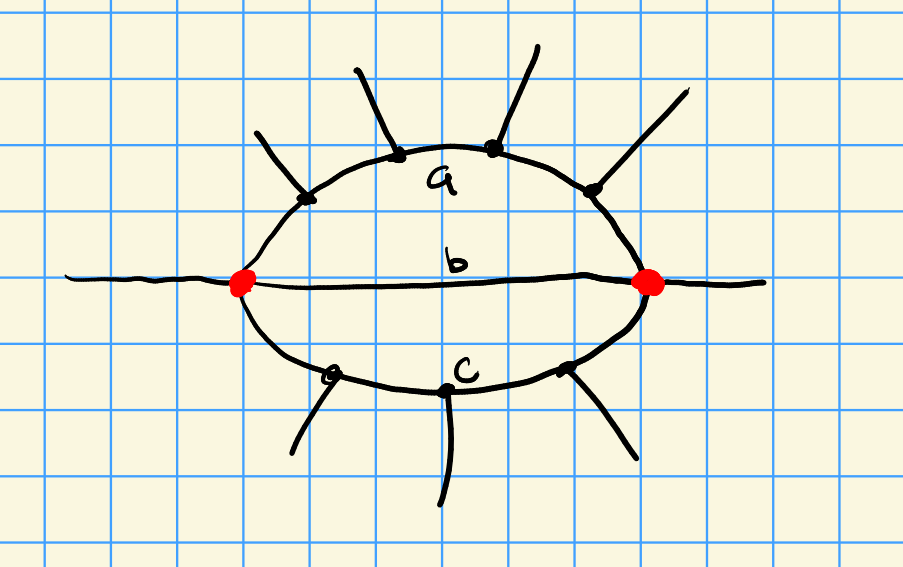What does the mixed Hodge structure on cohomology look like? This is an important step toward understanding periods, and the answer depends heavily on $$D$$. Bloch works out kite and double box graphs.

For $$(a,1,1)$$ hypersurfaces, identifies when certain homology (or some of its weight-filtered pieces) are Hodge-Tate under conditions on $$a$$ and $$D$$.

Note that a Tate HS has $$h^{i, j} = 0$$ for $$i\neq j$$, and mixed Tate is an iterated extension of such a Hodge structure. This means it’s as simple as it could reasonably be. Idea of proof: blow up a linear subspace, remove some singular hyperplanes.

Interestingly, for $$D=4$$ only the cases $$(3,1,1)$$ and $$(1,1,1)$$ yields a non-Hodge-Tate mixed Hodge structure. These correspond to multiple polylogarithms.

WIP: can say more in $$(a,1,c)$$ case when $$a, c> 1$$. Note that the $$(a,1,c)$$ case can’t yield K3s.

See Prym varieties?

# 11 Noriko Yui, Modularity of certain Calabi-Yau threefolds over $${\mathbb{Q}}$$ (Wednesday, July 20)

Definition: smooth projective variety $$X$$ over $${\mathbb{C}}$$, $$\dim_{\mathbb{C}}X = 3$$, and

• $$H^i(X; {\mathcal{O}}_X) = 0$$ for $$i=1,2$$, and
• $$K_X \cong {\mathcal{O}}_X$$.

By conjugation, $$h^{i, j} = h^{j, i}$$, and by Serre duality $$h^{i, j} = h^{3-i, 3-j}$$. Note $$h^{1,1}, h^{2,2} > 0$$ since Calabi-Yau threefolds are Kähler. The outer edge of the Hodge diamond contains only zeros and ones and has 7 rows, up to 4 columns. A typical example: quintic threefolds $$\sum x_i^5 = 0$$, or elliptic curves are dimension 1 CYs and K3s are dimension 2 CYs. More generally, CYs can be constructed as intersection threefolds in weighted projective spaces. Other examples:

• Double octics $$w^2 = f_8(x,y,z,w)$$
• $$E\times S/\iota$$ where $$E$$ is an elliptic curve, $$S$$ is a K3, $$\iota$$ is a non-symplectic involution
• Toric CYs

Let $$X_{/ {{\mathbb{Q}}}}$$ be Calabi-Yau iff $$X\otimes_{\mathbb{Q}}{\mathbb{C}}$$ is Calabi-Yau in the usual sense. Suppose it has defining equations in $${\mathbb{Z}}{ \left[ { \frac{1}{m} } \right] }$$ for some $$m$$, pick a good prime $$p$$ coprime to $$m$$ so that $$X$$ has good reduction: $$X_p$$ is smooth over $$\mkern 1.5mu\overline{\mkern-1.5mu{\mathbb{F}}_p\mkern-1.5mu}\mkern 1.5mu$$. Define the zeta function $$Z_p(X, T) \in {\mathbb{Q}}{\left[\left[ T \right]\right] }$$ as usual, then the Weil conjectures hold for $$X$$. Since $$P_1 = P_5 = 1$$, \begin{align*} Z_p(X, T) = {P^3(T) \over (1-T) P^2(T) P^2(pT) (1-p^3 T)} .\end{align*} There is a compatible system of $$\ell{\hbox{-}}$$adic Galois representations on étale cohomology. Define an $$L{\hbox{-}}$$function $$L_i(X, s) = \prod_{p\neq \ell \text{ good}} P^i(p^{-s})^{-1}\times (\cdots)$$, where the last factor accounts for when $$\ell=p$$. Can compute $${\sharp}X({\mathbb{F}}_p)$$ using the Lefschetz trace formula for $$\operatorname{Frob}_p\curvearrowright H_\text{ét}(\mkern 1.5mu\overline{\mkern-1.5muX\mkern-1.5mu}\mkern 1.5mu; { {\mathbb{Q}}_\ell })$$. Here $${\sharp}X({\mathbb{F}}_p) = 1 + p^3 + (1+p ) t_2(p) - t_3(p)$$ where $$t_2, t_3$$ are bounded above by $$h^{1, 1}, \beta_?$$ by RH.

The modularity question: are there global functions or automorphic forms that determine $$L(X, s)$$? This would be the Langlands philosophy.

Say $$X$$ is rigid if $$h^{2, 1} = 0$$ so $$\beta_3 = 2$$ and the Hodge diamond only has two unknowns, $$h^{1, 1}, h^{2,2}$$. These resemble elliptic curves in terms of the characteristic polynomial.

Every rigid Calabi-Yau threefold is modular: there is some modular form $$f$$ of weight $$4$$ on some $$\Gamma_0(N)$$ such that $$L(f, s) = L(X, s)$$.

There are 14 one-parameter families of CYs defined over $${\mathbb{Q}}$$ of hypergeometric type, and at conifold points each family as a rigid motive and corresponds to a special cusp form of weight 4 on some $$\Gamma_0(N)$$ where only $$2,3,5\divides N$$. The next simplest case is when $$h^{2, 1} = h^{1, 2} = 1$$ and not zero, so $$\beta_3 = 4$$. Constructing such CYs: Nygaard and van Geemen take a certain complete intersection whose singular locus has 16 ordinary double points and 4 plane conics intersecting in a square; take its resolution. Van Geemen and Werner/Consani and Scholten construct another using the affine equation of a skew pentagon, yielding a quintic Calabi-Yau threefold defined over $${\mathbb{Q}}$$ with bad primes $$2,3,5$$.

For $$X$$ the Calabi-Yau coming from a complete intersection as above, \begin{align*} L(X, s) = L(\chi^3, s) \cdot L(\chi_{(-1)}, s) ,\end{align*} where $$\chi$$ is a Hecke character for $${\mathbb{Q}}(i)$$?

Note that something similar happens for elliptic curves with CM. Idea of proof: split $$H^3$$ into two rank 2 motives to decompose the L function.

Since $$X$$ is covered by some $$E^3$$ where $$E: y^2 = 1+x^4$$ which has CM by $${\mathbb{Q}}(i)$$, so $$L(X, s) \divides L(E^3, s)$$ and we can use this to define when a Calabi-Yau has CM in terms of the cover. General expectation: if $$X$$ has CM for an imaginary quadratic number field $$K$$, then $$L$$ splits into factors involving Hecke characters of $$K$$. To avoid Hecke characters, define real multiplication instead: if $$X$$ is Calabi-Yau with $$h^{p, q} = 1$$ for $$p+q=3$$ and $$\beta_3 = 4$$, set $$K = {\mathbb{Q}}(\sqrt d)$$ and say $$X$$ has RM with $$K$$ if

• There is an algebraic correspondence $$\Psi:X\to X$$ defined over $$K$$.
• There is an automorphism of $$H^3$$ preserving the Hodge structure,
• There is an automorphism of $$H^3$$ preserving Galois representations.

See Hilbert modular forms, Adrianov $$L{\hbox{-}}$$functions.

# 12 Johannes Walcher, Rationality of MUMs and 2-functions (Thursday, July 21)

## 12.1 Rational 2-functions are abelian

Motivations: mirror symmetry links enumerative geometry (A model) to variations of Hodge structure (B model). On the B side, arithmetic properties naturally arise. Do they have an interpretation on the A side?

Classical mirror symmetry involves maximal unipotent monodromy (MUM) in the moduli space of CYs. Johannes entered this area by looking at extensions of algebraic cycles.

2-functions: for $$s \in {\mathbb{Z}}_{>0}$$, and $$s{\hbox{-}}$$function is a power series $$W\in z{\mathbb{Q}}{\left[\left[ z \right]\right] }$$ of the form $$\sum_d n_d \mathrm{Li}_s(z^d)$$. Motivating examples: $$g=0$$ prepotential expanded around MUM in flat coordinates. Equates generating functions for GW invariants and that for BPS invariants, $$\sum a_dq^d = \sum n_d \mathrm{Li}_3(q^d)$$.

A new feature: framing, where $$Y \in 1 + z{\mathbb{Z}}{\left[\left[ z \right]\right] }$$ defined an automorphism of $$z{\mathbb{Z}}{\left[\left[ z \right]\right] }$$. See Lagrange inversion for finding coefficients of power series. Theorem: if $$W$$ is a rational 3-function, then all of its framings are 3-functions. Relates to some classical theorems, e.g. the Wolstenholme theorem and the Jacobsthal-Kazandzidis congruence.

Over a number field, the $$s{\hbox{-}}$$functions form a finite rank free module. Muller shows that there can’t be a natural choice of basis for $$K$$ an arbitrary number field – if $$W\in zK{\left[\left[ z \right]\right] }$$ is rational 2-function, so $$\exp(\delta W) \in K(z)$$, then $$K/{\mathbb{Q}}$$ must be an abelian extension.

## 12.2 MUM

For $$\pi: Y\to B$$ a smooth family of Calabi-Yau threefolds of mirror quintic type, there is a $${\mathbb{Z}}{\mathrm{VHS} }$$ (pure, weight 3). By analog with $$p{\hbox{-}}$$adic Hodge theory, Kontsevich et al have a theorem that lets you conclude integrality from rationality in some special cases? Look at monodromy of Picard-Fuchs equations. See Bogner’s “strange operator.”

Early 2000s: Almkvist-Zudilin look at Calabi-Yau differential operators, which are 4th order operators with MUM points, duality, integral fundamental periods, etc so that it could come from the integral cohomology of a CY.

See Beauville-Apery families of elliptic curves, Hadamard product.

Idea: take a family $$Y\to B$$ and $$C\hookrightarrow Y$$ some 2-cycles (curves) which is also a nice family over $$B$$, this gives an extension of VHSs. Somehow classified by intermediate Jacobian? By Griffith’s transversality, the extension is fully determined by a piece in a certain $$\operatorname{Ext} ^1$$ group. Extraction of integers appears to depend on finding a good basis for 2-functions. Speculation: analytic 2-functions with coefficients in a number field arise as solution to inhomogeneous linear ODEs with algebraic coefficients, and unravelling the enumerative interpretation (e.g. in in open GW/BPS theory) will involve natural such bases. Maybe $$K$$ is the trace field of a hyperbolic 3-manifold?

# 13 Matt Kerr, $$K_2$$ and quantum curves (Thursday, July 21)

Holy moly, Matt has a lot of equations and writes super fast! I was not able to record even half of what was written.

## 13.1 A miraculous coincidence

Suppose $$f\in \operatorname{Mero}({\mathbb{C}}, {\mathbb{C}})$$ with no poles in the horizontal strip $${\left\lvert {\Im(z)} \right\rvert} < 2\pi$$, then $$e^{\pm 2\pi i {\frac{\partial }{\partial z}\,}}$$ is a shift operator $$f(z) \mapsto f(z+2\pi i)$$. Example: $$e^{2\pi i {\frac{\partial }{\partial z}\,}} z^k = \sum_\ell \qty{ (2\pi i)^\ell \over \ell! } \qty{{\frac{\partial }{\partial z}\,}}^\ell z^k = (z+ 2\pi i)^k$$. Consider \begin{align*} \widehat{\phi }\mathrel{\vcenter{:}}= e^r + e^{-r} + e^{2\pi {\frac{\partial }{\partial r}\,}} + e^{-2\pi i {\frac{\partial }{\partial r}\,} }\curvearrowright L^2({\mathbb{R}}) .\end{align*} This is some kind of quantum Hamiltonian for a Fermi gas? Note that this is unbounded, but its inverse is bounded and self-adjoint with spectrum that can be enumerated. This suggests a curve of the form $$x+x^{-1}+y+y^{-1}= \lambda$$, is there any AG or Hodge theory arising in this spectral problem?

Consider elliptic curves, pullbacks of the Legendre family $$y^2 = x(x-1)(x-16t^2)$$. This has two periods $$A(t), B(t)$$ given by integrating over the two torus cycles $$\alpha,\beta$$, which are complicated but annihilated by the Picard-Fuchs operator $$L = D_t^2 - 16t^2 (D_t + 1)^2$$. Solve $$L({-}) = c$$ a constant using variation of parameters to get \begin{align*} C(t) \approx B(t) \int A(t) {\,dt\over t} - A(t) \int B(t) {\,dt\over t} .\end{align*} Can choose constant terms such that the monodromy of $$C$$ lies in $${\mathbb{Z}}(2) \left\langle{A(t), B(t)}\right\rangle$$ where $${\mathbb{Z}}(2) = 2\pi i {\mathbb{Z}}$$. Set $$\nu(t) = {1\over \pi^2} \log^2(-t) + {7\over 6} + { \mathsf{O}} (t\log(t))$$ and define $$t_n$$ by $$\nu(t_n) = n+2$$. Then $$-\log(-t_n) = \pi\sqrt{n + {5\over 6}}$$, which yields a sequence matching the exponents in the eigenvalues of $$\widehat{\phi}$$. Something is going on!

Local mirror symmetry conjecture linking inhomogeneous Picard-Fuchs equations of Hori-Vafa models and enumerative geometry of local CYs: the $$B$$ model mirror of $$K_{{\mathbb{P}}^1\times {\mathbb{P}}^1}$$ is the $$\mathrm{VMHS}$$ of $$H^3$$ of $$Y_a = V(x+x^{-1}+ y+y^{-1}+ a + uv) \subseteq ({\mathbb{C}^{\times}})^2\times \times {\mathbb{C}}^2$$ with coordinates $${\left[ {x, y} \right]}$$ and $${\left[ {u, v} \right]}$$. See Frobenius duality for differential operators? The Geneva conjectures connected difference operators and quantum curves to enumerative geometry of CYs.

## 13.2 Beilinson’s Formula

Like $$X_{/ {{\mathbb{C}}}}$$ smooth projective, and note \begin{align*} {\mathsf{K}}_2({\mathbb{C}}(X)) = { {\mathbb{C}}(X)^{\times}\wedge {\mathbb{C}}(X)^{\times}\over \left\langle{F \wedge (1-F)}\right\rangle } \xrightarrow{\text{T}} \bigoplus _{p\in ?} {\mathbb{C}^{\times}} \end{align*} where $$T$$ is the tame symbol. We want to compute the regulator \begin{align*} \ker(T) = H_M^2(X, {\mathbb{Z}}(2)) \xrightarrow{R_X} H^2_D(X, {\mathbb{Z}}(2)) \cong H^1(X^{\mathrm{an}}, {\mathbb{C}}/{\mathbb{Z}}(2)) .\end{align*} Beilinson shows that if $$\left\{{f, g}\right\}\in \ker(T)$$ and $$\gamma\in \pi_1(X\setminus?, x_0)$$ then \begin{align*} {\left\langle {R_X\left\{{f, g}\right\}},~{[\gamma]} \right\rangle} \equiv \int_\gamma \log(f) {dg\over g} - \log g(x_0) \int_\gamma {df\over f} .\end{align*}

Consider $$X \xrightarrow{\pi} S$$ a family of smooth curves and $$z\in H_m^2(X, {\mathbb{Z}}(2))$$ we can consider their fibers $$z_2\in H_M^2(X_s, {\mathbb{Z}}(2))$$. This maps to \begin{align*} R(S) = H^1(X_s, {\mathbb{C}}/{\mathbb{Z}}(2)) \cong \operatorname{Ext} ^1_{\mathrm{MHS}}({\mathbb{Z}}(2), H^1(X_s, {\mathbb{Z}})) .\end{align*} By Griffiths transversality, $$\nabla R = F^1 H^1_{X_{/ {S}} } \otimes\omega_S^1$$. If $$z$$ lifts to $$\left\{{F, G}\right\}$$ (?) then $$\nabla R = {dF\over F}\wedge {dG\over G}$$.

## 13.3 Families of elliptic curves

Let $$\Delta \subseteq {\mathbb{R}}\times [-1, 1]$$ be a reflexive polytope, e.g. the polytope for $${\mathbb{P}}^n$$, let $$\Delta^\circ$$ be is polar dual. Somehow construct an elliptic surface $${\mathcal{E}}$$ out of this! The symbol $$\left\{{-x, -y}\right\}\in {\mathsf{K}}_2({\mathbb{C}}({\mathcal{E}}))$$ lifting to $$z\in H^2_M(\Sigma\setminus{\mathcal{E}}_\infty, {\mathbb{Z}}(2))$$ and take its regulator in $$H^1(E_?, {\mathbb{C}}/{\mathbb{Z}}(2))$$.

Idea: regulators of $${\mathsf{K}}_2$$ of curves relate to Hori-Vafa models, so we’ve reduced to relating regulators to quantum curves: